## Dirty Map

From the van Cittert-Zernicke theorem,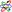the relationship between observed visibility functionand source brightnessin synthesis imagingis given by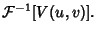(1)

But the visibility functionis sampled only at discrete points(finite sampling), so only an approximation to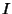, called the dirty map'' and denoted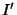, is measured. It is given by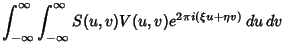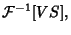(2)

whereis the sampling function andis the observed visibility function.Letdenote Convolution and rearrange the Convolution Theorem,(3)

into the form(4)

from which it follows that(5)

Now note that(6)

is the CLEAN Map, and define the Dirty Beam'' as the inverse Fourier Transform of the sampling function,(7)

The dirty map is then given by(8)

In order to deconvolve the desired CLEAN Mapfrom the measured dirty mapand the known Dirty Beam, the CLEAN Algorithm is often used.